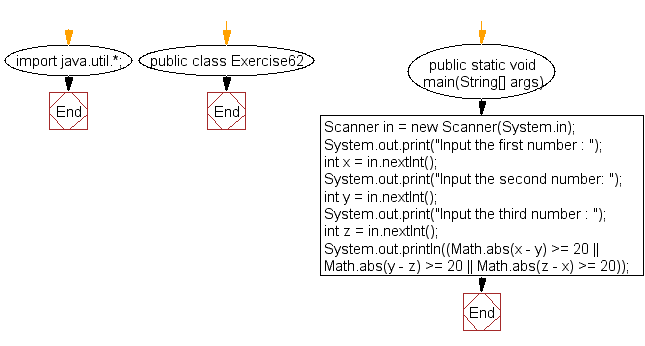﻿ Java: If one of the 3 integers is 20 or higher and less# Java Exercises: Accepts three integer values and return true if one of them is 20 or more and less than the substractions of others

## Java Basic: Exercise-62 with Solution

Write a Java program that accepts three integer values and return true if one of them is 20 or more and less than the substractions of others.

Sample Solution:

Java Code:

``````import java.util.*;
public class Exercise62 {
public static void main(String[] args)
{
Scanner in = new Scanner(System.in);
System.out.print("Input the first number : ");
int x = in.nextInt();
System.out.print("Input the second number: ");
int y = in.nextInt();
System.out.print("Input the third number : ");
int z = in.nextInt();
System.out.println((Math.abs(x - y) >= 20 || Math.abs(y - z) >= 20 || Math.abs(z - x) >= 20));
}
}
```
```

Sample Output:

```Input the first number : 15
Input the second number: 20
Input the third number : 25
false
```

Pictorial Presentation:Flowchart:Java Code Editor:

What is the difficulty level of this exercise?

Test your Programming skills with w3resource's quiz.

﻿

## Java: Tips of the Day

countOccurrences

Counts the occurrences of a value in an array.

Use Arrays.stream().filter().count() to count total number of values that equals the specified value.

```public static long countOccurrences(int[] numbers, int value) {
return Arrays.stream(numbers)
.filter(number -> number == value)
.count();
}
```

Ref: https://bit.ly/3kCAgLb